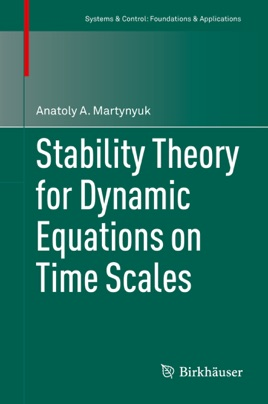• USD 89.99

## Descripción de editorial

This monograph is a first in the world to present three approaches for stability analysis of solutions of dynamic equations. The first approach is based on the application of dynamic integral inequalities and the fundamental matrix of solutions of linear approximation of dynamic equations. The second is based on the generalization of the direct Lyapunovs method for equations on time scales, using scalar, vector and matrix-valued auxiliary functions. The third approach is the application of auxiliary functions (scalar, vector, or matrix-valued ones) in combination with differential dynamic inequalities. This is an alternative comparison method, developed for time continuous and time discrete systems.In recent decades, automatic control theory in the study of air- and spacecraft dynamics and in other areas of modern applied mathematics has encountered problems in the analysis of the behavior of solutions of time continuous-discrete linear and/or nonlinear equations of perturbed motion. In the book “Men of Mathematics,” 1937, E.T.Bell wrote: “A major task of mathematics today is to harmonize the continuous and the discrete, to include them in one comprehensive mathematics, and to eliminate obscurity from both.”Mathematical analysis on time scales accomplishes exactly this.
This research has potential applications in such areas as theoretical and applied mechanics, neurodynamics, mathematical biology and finance among others.